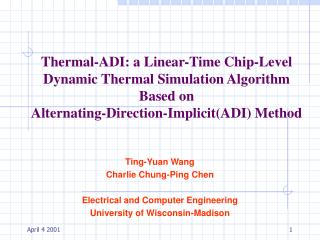Download PresentationTing-Yuan Wang Charlie Chung-Ping Chen Electrical and Computer Engineering

# Ting-Yuan Wang Charlie Chung-Ping Chen Electrical and Computer Engineering - PowerPoint PPT PresentationDownload Presentation## Ting-Yuan Wang Charlie Chung-Ping Chen Electrical and Computer Engineering

- - - - - - - - - - - - - - - - - - - - - - - - - - - E N D - - - - - - - - - - - - - - - - - - - - - - - - - - -
##### Presentation Transcript

1. Thermal-ADI: a Linear-Time Chip-Level Dynamic Thermal Simulation Algorithm Based on Alternating-Direction-Implicit(ADI) Method Ting-Yuan Wang Charlie Chung-Ping Chen Electrical and Computer Engineering University of Wisconsin-Madison

2. Motivation • 1999 International Technology Roadmap for Semiconductor (ITRS) • Maximum power • Number of metal layers • Wire current density

3. 1999 ITRS

4. Existing Thermal Simulation Methods • Finite Difference Method • Easy, good for regular geometry, fast • Finite Element Method • More complicated, good for irregular geometry • Equivalent RC Model (S.M. Kang) • Compatible with SPICE model, need to solve large scale matrix

5. Finite-Difference Formulation of the Heat Conduction on a Chip • Space Domain • Time Domain

6. Heat Conduction Equation where : Temperature : Material density : Specific heat : Heat generation rate : Time : Thermal conductivity

7. Energy Conservation Increasing rate of stored energy which causes temperature increase Net rate of energy transferring into the volume Heat generation rate in the volume

8. Space Domain Discretization • Heat Conduction Equation • Central-Finite-Difference Approximation

9. Time domain discretization • Heat Conduction Equation • Simple Explicit Method • Simple Implicit Method • Crank-Nicolson Method

10. Simple Explicit Method • Accuracy: • Stability Constraint: • No matrix inversion but time steps are limited by space discretization

11. Simple Implicit Method Accuracy: Unconditionally Stable No limits on time step but involves with large scale matrix inversion

12. Crank-Nicolson Method Accuracy: Unconditionally stable No limits on time step but involves with large scale matrix inversion

13. Analysis of Crank-Nicolson Method e.x. m=4,n=4 Total node number N = mn n m Matrix size = NxN

14. Alternating Direction Implicit Method Solves higher dimension problem by successive Lower dimension methods Accuracy: Unconditionally stable No limits on time step and no large scale matrix inversion

15. Alternating Direction Implicit Method Step I: x-direction implicit y-direction explicit Step II: x-direction explicit y-direction implicit n • Peaceman-Rachford Algorithm • Douglas-Gunn Algorithm

16. Peaceman-Rachford Algorithm • Step I • Step II

17. Step I Step II Douglas-Gunn Algorithm

18. Illustration for ADI Step I Step II X-direction implicit Y-direction implicit n n … … 2 2 j = 1 j = 1 i = 1 2 … m 1 2 … m

19. Analysis of ADI Method X-direction implicit Tridiagonal Matrix n … 2xnxm = 2nm =2N 2 2 steps n matrices tridaigonal matrix j = 1 i = 1 2 … m Time complexity: O(N)

20. Three Different Locations of Node (case I) (case II) (case III) (i,j+1) (i,j+1) (i,j+1) Si Si Heat Source Heat Source Heat Source (i-1,j) (i,j) (i+1,j) (i-1,j) (i,j) (i+1,j) (i-1,j) (i,j) (i+1,j) (i,j-1) (i,j-1) (i,j-1)

21. Results comparison

22. Result – Run Time Comparison II

23. Results – Memory Usages I

24. Results – Memory Usages II

25. Results – Stability Constraint Gamma is the stability limit for simple explicit method

26. Thank you for your attention!